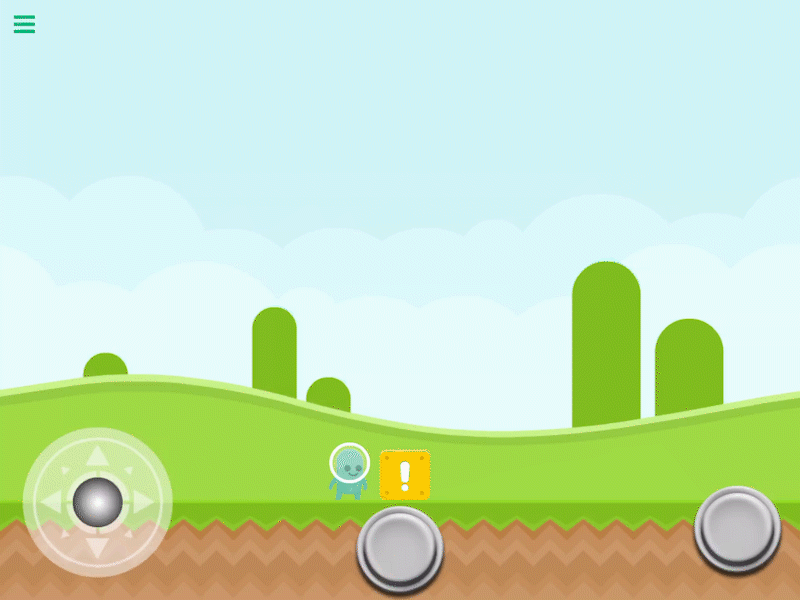# Propel Object

Description

The Propel Object behavior will apply a force so that two selected objects move away from each other.

This behavior will Trigger an event immediately.

Properties

 Object A This is one of the objects you want to propel.The default properties will result in this object staying stationary and Object B being moved away from Object A. By default this is the current object selected. You can change this to any other object in your scene. Object B This is the second object you want to propel. The default properties will result in this object moving away from Object A. Anchor This is the specific X and Y location on the objects where the the force will be applied. Eg, the hand. on your character. You can enter the numbers manually, or visually by moving the blue target when selecting Object A or Object B from the behavior properties. Force X This is the amount of force to apply to each object. Force is measured in newtons. Object A Multiplier This will multiply the force on Object A away from Object B by a specified amount. The default value is 0, meaning Object A will have no force applied to it and will stay stationaryIf this value is 1, and Object B multiplier is 0 then Object A will move away from Object B. Object B Multiplier This will multiply the force on Object B away from Object A by a specified amount. The default value is 1, meaning it will move away from Object A.If this value is 0, and Object A multiplier is 1 then Object A will move away from Object B.

Outputs

 Object A ID This outputs the Object ID of Object A. Object B ID This outputs the Object ID of Object B.

Examples

You can use this to simulate an explosion so your object moves away from each other. When used with tags, you can move multiple objects away from Object A which would be the center of the explosion